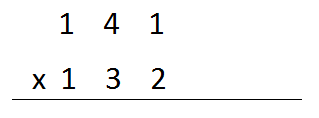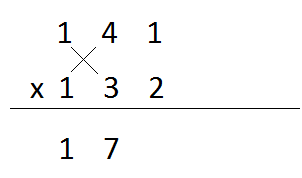Multiplication- as it should be done (Vedic math)

Ever been thwarted by a math exam because of your slow or inaccurate multiplication, even when u know your trigonometry ratios. Here’s a method to uncomplicate your life and save you the invaluable time in your math exam

Principle:

This method mainly states multiply diagonally wherever possible and if not, multiply vertically

Brief history (as if you wanna know😏!):

This method was obtained from the Sanskrit Vedas, and therefore is considered a part of Vedic math but you don’t want me babbling about its history, so here is the method…

Lengthy Tutorial:

1)      You would first want to take out a piece of paper 😛
Ok fine, I get it… for real now
1)      For this example, we’re going to multiply 141 and 1322)      Then we’re going to consider the leftmost digit of both the numbers and multiply them. In this case 1x1. Write the product i.e. 1, underneath. Like this:3)      Next, we take the 2 leftmost digits of both the numbers and multiply them diagonally, add both the products and write it alongside 14)      Now we consider all the digits, multiply the extremes diagonally, and multiply the middle digits vertically, and add all 3 products. As the sum is 15 we write the 1 subscripted to the 7 and the 5 underneath.5)      Now we consider the digits at the ones and the tens unit of both the numbers and multiply them diagonally and do the usual (you must’ve got the hang of it by now).6)      Now we consider the rightmost digits and multiply them vertically7)      Now you got to add the carry overs. And voila!! You got your answer. Simple, right?Same principle for a different number of digits… multiply diagonally whenever possible and vertically when not possible. This method also has another benefit. It gives you the most valued digit first, which can help you estimate and works a wonder in competitive exams!

Some words of caution:-

·        Take care of the place values. Tens should be under tens, hundreds under hundreds,
·        Make sure you add the products. Not multiply them.
you find it tedious to calculate the square root of numbers? Think its too long of a method? Here's something that might help!!!
This is my first article here, hope you guys like it. Comment below for help or what you wanna see me write next. Reviews are highly appreciated. Stay tuned for more awesome work from me…

Have a nice day showing off your super mathematical powers to your friends